Thank you for visiting nature.com. You are using a browser version with limited support for CSS. To obtain the best experience, we recommend you use a more up to date browser (or turn off compatibility mode in Internet Explorer). In the meantime, to ensure continued support, we are displaying the site without styles and JavaScript.

# Ultrasensitive magnetic field detection using a single artificial atom

## Abstract

Efficient detection of magnetic fields is central to many areas of research and technology. High-sensitivity detectors are commonly built using direct-current superconducting quantum interference devices or atomic systems. Here we use a single artificial atom to implement an ultrasensitive magnetometer with micron range size. The artificial atom, a superconducting two-level system, is operated similarly to atom and diamond nitrogen-vacancy centre-based magnetometers. The high sensitivity results from quantum coherence combined with strong coupling to magnetic field. We obtain a sensitivity of 3.3 pT Hz−1/2 for a frequency at 10 MHz. We discuss feasible improvements to increase sensitivity by one order of magnitude. The intrinsic sensitivity of this detector at frequencies in the 100 kHz–10 MHz range compares favourably with direct-current superconducting quantum interference devices and atomic magnetometers of equivalent spatial resolution. This result illustrates the potential of artificial quantum systems for sensitive detection and related applications.

## Introduction

Sensitive physical measurements are an essential component of modern science and technology. In particular, detection of magnetic fields is an area of wide interest, with various applications including medical imaging, geomagnetics, non-destructive materials evaluation, scanning probe microscopy, and electrical measurements1,2. Magnetic field sensors are also enabling tools for fundamental studies of magnetism3, spin dynamics4, fundamental symmetries2 and mechanical motion5,6.

Sensitive tools to detect magnetic fields are diverse and include direct-current superconducting quantum interference devices (DC-SQUIDs)1, atomic magnetometers2, Hall probes7 and magnetostrictive sensors8. DC-SQUIDs have been established for a long time as very sensitive magnetometers1. In recent years, significant advances in atomic control led to the development of atomic magnetometers, which presently compete with DC-SQUIDs for magnetic field detection and have the convenience of operation in a room temperature environment2. Atomic magnetometers rely on the measurement of the effect of the field on the coherent dynamics of an ensemble of atoms. A detection method similar to atomic magnetometry can be implemented using nitrogen-vacancy defects (NV centres) in diamond crystals9. Magnetometers based on single NV centres10,11 have been demonstrated and shown to have interesting prospects as high-spatial-resolution high-sensitivity detectors.

The principle of our approach follows closely that of magnetometry based on vapour cells or NV centres2,9. The method employs a Ramsey interferometry sequence. The first step is initialization of the spin in a superposition of the up and down states with respect to a reference axis. Precession due to an applied magnetic field for a duration τ around time t leads to an accumulated phase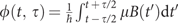, with μ the magnetic moment and B(t) the time-dependent component of the magnetic field along the reference axis. Next, the spin is rotated with a coherent control pulse, so that the projection along the reference axis depends on φ(t, τ), hence on the magnetic field. A single measurement produces a result r=±1, corresponding to the two spin states. For N repetitions of the sequence, the average value of the measurement 〈r〉=sinφ and the standard deviation is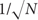. The minimum detectable magnetic field difference, corresponding to a signal to noise ratio of 1, is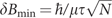. This can be expressed as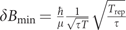, with Trep the repetition time of the procedure described above and T=NTrep the total measurement time. The sensitivity can thus be expressed as the quantity δBmin, which has units of T Hz−1/2. The sensitivity increases with the time τ as long as the evolution is fully coherent; with decoherence taken into account, the optimum is reached when τ is of the order of the Ramsey coherence time T2*.

In existing magnetometers based on this principle, the two spin states of interest are hyperfine or Zeeman levels in alkali atoms2 or spin sublevels of the ground state in NV centres in diamond9. Detection is done most commonly using an ensemble of atoms/defects. With Nat atoms, sensitivity is further enhanced by a factor=the volume and n the density of the cloud. Thedependence of sensitivity implies that there is a tradeoff between sensitivity and spatial resolution. The same tradeoff also appears in magnetic field sensors based on DC-SQUIDs1.

In this paper, we demonstrate ultra-high sensitivity detection of alternating current (AC) magnetic fields using a single artificial atom. The artificial atom is a persistent current qubit (PCQ)—a micron-sized superconducting ring with Josephson junctions12. This system has been studied extensively for quantum computing applications13. Owing to the interaction with its solid state environment, the PCQ has a coherence time that is shorter than in typical atomic systems. The lower coherence time is unfavourable for sensitive detection. However, this drawback is compensated for by the large coupling to magnetic field, typically five orders of magnitude larger than in atomic systems. To optimize magnetic field detection sensitivity, we use an operation mode based on detection of correlation of qubit measurement results. We find a sensitivity of 3.3 pT Hz−1/2 for operation at 10 MHz. The detection bandwidth around a centre frequency of 8 MHz reaches 1 MHz, limited at present by the overhead time introduced by measurement. The measured sensitivity is comparable with state of the art magnetometers based on DC-SQDUIDs or atoms/NV centres of equivalent spatial resolution. We present a theoretical analysis which shows that one order of magnitude further improvement is possible with feasible changes in the detection scheme. Our results demonstrate the potential of artificial quantum systems for high-sensitivity low-backaction detection.

## Results

### Coherent control and measurement of the qubit state

The artificial atom used in our experiments for magnetic field detection, a PCQ, is a superconducting loop with three Josephson junctions, shown in Fig. 1. The qubit is operated in a dilution refrigerator at a temperature of 43 mK. The qubit Hamiltonian is given by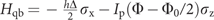, where Φ is the magnetic flux applied to the qubit ring, Φ0=h/2e is the flux quantum, and hΔ is the minimum energy-level splitting of the qubit, which occurs at the symmetry point Φ=Φ0/2 (see Fig. 1d). This representation of the Hamiltonian uses two states with persistent current ±Ip flowing in the qubit ring. The effective magnetic moment of the qubit is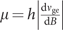with ge the energy-level difference between the excited (e) and ground (g) states and B=Φ/Aqb the magnetic field applied to the qubit ring; Aqb is the qubit loop area. The magnetic moment is given by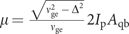. For our qubit, characterized by Ip=140 nA, Aqb=24.5 μm2 and Δ=9.82 GHz, and operated at νge=11.39GHz, μ reaches the value 3.8 × 105 μB. This large magnetic moment enables a large sensitivity, despite the coherence time of the PCQ being shorter than in typical atomic systems2,9. We note that a different method to use a PCQ for magnetometry, based on susceptibility of a qubit as opposed to quantum coherent dynamics, was discussed in 14; the predicted sensitivity is lower than for the detection scheme we discuss here.

Control of the state of the PCQ is done by an applied magnetic flux Φ. We bias the qubit with a flux Φ(t)=Φb+δΦ(t), where Φb is a static bias flux produced by an external coil and δΦ(t) is a fast varying flux coupled to the qubit via a coplanar waveguide terminated by an inductance (see Fig. 1a). We can write the qubit Hamiltonian as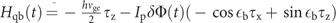, where τx and τz are Pauli operators in the energy eigenbasis and ɛb=arctan(Ipb−Φ0/2)/(hΔ)). Coherent qubit control is implemented by applying a flux δΦ(t)=δΦ0(t)cos(2πνgetβ(t)) with the amplitude δΦ0(t) and the phase β(t) slow (compared with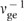) functions of time. In the frame rotating at the qubit frequency, the Hamiltonian is given by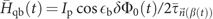. Here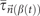is the Pauli operator along an axis in the xy plane at an angle β(t) with the x axis. Below, we use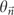to denote a rotation of angle θ around an axis defined by the vector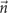.

Quantum measurement of the PCQ state is performed by using a circuit-quantum electrodynamics set-up in the dispersive regime15,16,17,18. The qubit is inductively coupled to a superconducting resonator, with a resonance frequency νres=6.602 GHz, and quality factor Q=4,000 defined by coupling to the input and output ports. The resonance frequency of the cavity is modified due to the presence of the qubit by an amount that depends on the state of the qubit. Consequently, the complex transmission coefficient at frequencies close to the resonance frequency νres is strongly qubit state dependent. To measure the state of the qubit, a microwave readout pulse of duration Tr and frequency νr=νres is sent to the resonator. The complex amplitude of the transmitted pulse, as determined in a homodyne measurement19, is averaged over the duration Tr of the readout pulse. In Fig. 2 we present the results of the qubit measurement. We only show one quadrature of the transmitted voltage, VH; the axis for this quadrature is chosen such that it optimizes the measured signal (see Fig. 1a). The qubit is prepared either in the ground state, by allowing for a waiting time much longer than the energy relaxation time T1=1.3 μs (Fig. 2a) or in the excited state by including a πx pulse before readout (Fig. 2b). The distribution of the values of the homodyne voltage VH is plotted in Fig. 2c and d, respectively. The distribution is bimodal, with the two modes corresponding to the qubit energy eigenstates. A threshold is used to separate the distribution into a part labelled r=−1 and the complementary part labelled r=1. The threshold is chosen so that it optimizes the readout contrast, which is the difference of the conditional probabilities P(r=−1|e)−P(r=−1|g). The maximum contrast is 66%. This high readout fidelity is essential for the sensitivity of the detector.

### Procedure for AC magnetic field detection

In typical magnetometers, as introduced above, free precession in a magnetic field is employed. This procedure is adapted to detection of low-frequency fields, with frequency ranging from DC to 1/2Trep, where Trep is the repetition time of the control/measurement procedure. For a PCQ operated in this way, the detection sensitivity is low due to the short Ramsey coherence time T2*, which is limited by low-frequency magnetic flux noise20,21. Low-frequency noise sets the ultimate limit for measurement of low-frequency fields, a situation also encountered for DC-SQUIDs1. For this reason, we focus here on detection of AC magnetic fields. The procedure is illustrated in Fig. 3a. The qubit is controlled using a series of spin-echo pulse sequences, with each sequence consisting of pulses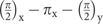applied at times tτ/2, t and t+τ/2, respectively. The qubit phase precession for the sequence at time t is given by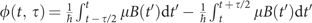(see Supplementary Fig. S1). The amplitude Bac of an AC field B(t)=Baccos(2πνst+α) can be detected optimally when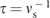and α=0. In general, applying pulses with fixed τ is sufficient to detect the two quadratures of a field at frequency τ−1 with a band ranging from DC up to bmax/2. The bandwidth bmax is smaller than τ−1 and further limited by 1/Trep when sampling issues are taken into account (see Supplementary Fig. S2 and Supplementary Methods). Therefore, the useful detection frequency band is (τ−1bmax/2, τ−1+bmax/2). The advantage of detection using spin-echo pulses as opposed to free precession is that the corresponding coherence time, T2, is significantly longer than the Ramsey time T2* (ref. 20), which renders the sensitivity to AC field higher than for low-frequency fields.

We focus first on experiments on detection at fixed frequency νs=τ−1, using the procedure shown in Fig. 3a. The magnetic field is induced by applying an AC voltage of amplitude Vac to the transmission line used for qubit control (see Fig. 1a) and acts via the term δΦ(t) in the qubit Hamiltonian Hqb(t). The resulting magnetic flux and field amplitudes are denoted by Φac and Bac, respectively. The phase accumulated during the spin-echo sequence is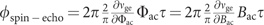(see Supplementary Methods). An oscillation in the qubit population versus time τ is expected, with a frequency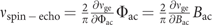. We indeed observe oscillations (see Fig. 3b) with a frequency proportional to Vac (see Fig. 3c).

### Field detection using qubit reset by energy relaxation

We next proceed to the characterization of magnetic field sensitivity. The output of the detector is the noisy binary signal r. The noise in r reflects the stochastic nature of quantum measurement. In the following, we express the results of detection in terms of the magnetic flux Φ applied to the qubit, as this facilitates the comparison with DC-SQUIDs. The noise in r can be represented as an equivalent noise in the amplitude Φac of flux oscillations. The latter is characterized by the spectral density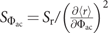. Here Sr is the single-sided spectral density of the noise in the qubit readout and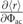is the transfer function of the detector, with 〈r〉 the average value of r. The transfer function can be expressed as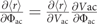. The factor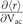is determined from the linear fit in Fig. 3d and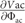is the ratio of the factor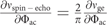determined from qubit spectroscopy and of the factor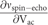determined from the data in Fig. 3c. In this way we determine the equivalent detector input noise fully from a set of measurable quantities, without any assumption on coupling of the field to the qubit. We note that 〈r〉≠0 at Vac=0, due to the measurement asymmetry (see histograms in Fig. 2c). We use a spin-echo control sequence time τ=100 ns chosen to correspond approximately to the optimal value for the measured qubit coherence time T2*. We determined T2=126±7 ns by fitting the temporal decay of the spin-echo oscillation envelope with the function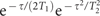, with T1 independently determined20. Figure 3e shows Sr and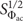for one value of Vac. We note that the noise depends very weakly on Vac as long as 〈r〉<<1, which is the case for all the detector operation conditions reported here. A theoretical calculation of the sensitivity taking into account the finite measurement fidelity and qubit decoherence predicts a flat noise spectrum with a value of (7.3±0.6) × 10−8 Φ0 Hz−1/2, in good agreement with the experimentally measured value of 8.9 × 10−8 Φ0 Hz−1/2 at high frequency. In the low-frequency region, excess noise is observed due to electronics drifts and interference. For fast detection, the equivalent flux noise is largely dominated by the value in the high frequency limit. For magnetic field detection, the sensitivity can be expressed as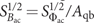, yielding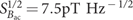.

### Field detection based on the correlation method

The detection sensitivity obtained above is limited primarily by the large ratio Trep/τ, which is in turn due to imposing Trep>>T1 in order to prepare the qubit by energy relaxation. To improve the detection efficiency, we use another method. We take as detector output not the series ri, but rather the series ci+1=ri+1ri. The usefulness of this method can be understood by considering the ideal case of projective measurements and very long relaxation time. If ri=1, ci+1=ri+1. If ri=−1, the qubit is prepared in the excited state; the probabilities to obtain results ri+1=1 and ri+1=−1 are reversed; this is compensated for automatically as ci+1=−ri+1. The correlator ci has the same statistical properties as ri in the previous method, which was based on qubit preparation by energy relaxation (see Supplementary Methods). The advantage is that the repetition time is significantly reduced. With qubit energy relaxation during measurement22,23 and finite projection fidelity, the effectiveness of this scheme is reduced, however, the lower repetition time makes the overall result significantly better. The repetition time of the sequence Trep is experimentally optimized to balance two competing effects: a long Trep results in additional qubit relaxation, which reduces qubit projection when r=−1; a short Trep leads to additional decoherence of the qubit, presumably due to photon number fluctuations in the resonator24. We analyse the data using a method fully analogous to the method discussed above, which employs qubit relaxation. The relevant data is shown in Fig. 3f. Similarly to the experiment presented in Fig. 3d, 〈c〉≠0 at Vac=0 is observed in Fig. 3f due to the measurement bias. A significant improvement of sensitivity is achieved compared with the result obtained using qubit reset by relaxation. The equivalent flux noise, taken as the average in the frequency range 1–100 kHz, is 3.9 × 10−8 Φ0 Hz−1/2. We note that a minor reduction in the noise power is observed at frequencies above 100 kHz, which was confirmed by numerical simulations to be related to the asymmetry of the measurement as induced by energy relaxation23. Excess noise is observed at low frequency, however, the magnitude is significantly lower than that for the data shown in Fig. 3e, due to the fact that low-frequency fluctuations in the detection system are removed by using the correlations. The magnetic field detection sensitivity reaches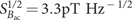.

### Detector frequency and amplitude response

We next present experiments on wideband detection using the correlation method (see Fig. 4). We applied magnetic fields at different frequencies νs, with a starting phase which is arbitrary at the beginning of each sequence. We extracted the noise power using the method explained above. For frequencies νs in the band (τ−1−(2Trep)−1, τ−1+(2Trep)−1), a peak is observed at frequency |νsτ−1| (see Fig. 4a). For νs outside this interval, a signal is detected for νs in a bandwidth τ−1 around centre frequency τ−1, however, undersampling will make this peak appear at a frequency translated to the detection band (see Supplementary Methods); this is the case for νs=8.990 MHz in Fig. 4a. We also characterized the amplitude response of the detector, at a frequency νs=8.05 MHz, which is offset with respect the central frequency τ−1 (Fig. 4b). The response is linear, with a slope compatible with the conversion factor determined based on spin-echo measurements for νs=τ−1 (see Supplementary Fig. S3). Finally, we performed an experiment in which we applied two tones at different frequencies, with the result shown in Fig. 4c. Additional experiments, characterizing the response to a change in the signal phase, are presented in Supplementary Fig. S4. The combination of experiments presented in this section, demonstrating amplitude, frequency and phase response, indicates that realistic signals can be measured using our detector.

## Discussion

These results establish the PCQ as an ultrasensitive AC magnetic field detector. In the following, a discussion is given on how our detector compares with other types of sensors. We focus on the comparison with DC-SQUIDs and atom-based detectors. For DC-SQUIDs, the most relevant figures of merit are the flux noise SΦ and the uncoupled energy sensitivity =SΦ/2L, with L the loop inductance. The latter is particularly relevant for magnetometry1. For the PCQ in this experiment,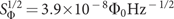and using L=27 pH we calculate. The SQUID in ref. 25, operated at 290 mK, had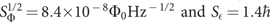. The SQUID in ref. 25, operated at 25 mK, had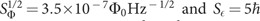. In both of these cases the energy sensitivity is the value measured at frequencies beyond kHz, where flux noise is negligible. The PCQ figures of merit in this experiment, which are still far from the theoretical limits that we discuss below, compare well with the optimized DC-SQUIDs in refs 25 and 26. We also note that optimization of the uncoupled energy sensitivity of the PCQ can be done by increasing the loop inductance, as flux noise was observed in general not to scale up with loop size20; this aspect is favourable for magnetometry1. The attained energy resolution and the prospects for further improvement, discussed below, raise interesting questions concerning the measurement backaction, as discussed for DC-SQUIDs in (refs 27 and 28).

For a comparison with atomic and NV centre-based detectors, the most suitable figure of merit is the quantity δBmin, which combines the sensitivity δBminand the detector volume V (ref. 2). Atomic magnetometers based on vapour cells have very high sensitivity, achieved usually with volumes of the order of 1 cm3, when sensitivities of the order of 0.1−1 fT Hz−1/2 (refs 3 and 29) are reached. When extrapolated to volumes of ≈1 μm3, corresponding to the PCQ detector used in this work, the ultimate theoretical limit to sensitivity is of the order of 1 pT Hz−1/2 (ref. 30). More recently, magnetic field detection based on cold atoms has been explored as well31,32. Using a Bose–Einstein condensate, a sensitivity of 8.3 pT Hz−1/2 was experimentally demonstrated in 31 for a measurement area of 120 μm2. The fundamental theoretical limit for sensing using a Bose–Einstein condensate with a resolution of a few micrometres33 is in the pT Hz−1/2 range. We note that atomic magnetometers operate typically at low frequency, below 1 kHz; methods exist to extend the operation frequency to hundreds of kHz (ref. 34). NV centres in diamond9 have recently emerged as an ultrasensitive method for magnetometry. They combine the possibility to work at room temperature and a spatial resolution that can be changed from the nanometre range (for single NV centre operation)10,11,35 up to large scale by using a spin ensemble. Decoherence due to paramagnetic impurities limits the flux detection efficiency to 0.250 fT Hz−1/2 cm3/2, optimal for AC fields at frequencies of the order of 100 kHz (ref. 9), which results in a sensitivity of 100 pT Hz−1/2 for a detection volume of the order of 1 μm3.

We discuss next the optimization of the detector sensitivity based on a set of realistic assumptions on parameters and feasible improvements of control and decoherence. At a given frequency νs, optimizing the detection sensitivity requires tuning of the magnetic moment of the qubit. This can be achieved in situ by changing the qubit transition frequency νge. The dependence of the optimal sensitivity on frequency is calculated with the following assumptions. First, the persistent current Ip and minimum energy-level splitting Δ are taken to have values as the PCQ in this experiment. Second, ideal projective measurements are used; projective measurements nearly reaching perfect fidelity have been demonstrated for superconducting qubits (see e.g., ref. 22). Third, the duty cycle (τ/Trep) is set equal to one. This can be achieved by shortening readout times, as enabled by nearly quantum-limited amplifiers36,37, and by replacing the spin-echo sequence with more complex control pulse schemes9,21,35. Last, qubit pure dephasing is assumed to be limited by 1/f flux noise (see ref. 20), with a spectral density given by (3.4 μΦ0)2/f[Hz], which is the value that would explain the observed spin-echo decay time at νge=11.39 GHz in our experiment, if flux noise was the only noise contribution. This level of flux noise is larger than measured values in smaller area superconducting rings20,21,38. It is very likely that in our experiment the flux noise is significantly lower than this upper bound and that charge noise has a major role, due to the low Josephson energy in this device. Straightforward changes in design will allow reducing the influence of charge noise to negligible levels. With these assumptions taken into account, the calculated optimal sensitivity (see Supplementary Fig. S5 and Supplementary Methods) is plotted in Fig. 5 for a PCQ with an energy relaxation time of 10 μs (attained in ref. 21) and for the case where relaxation is neglected. This calculation shows that with respect to the experimental results reported here, more than one order of magnitude of improvement is possible by feasible changes of the experimental set-up. The detection efficiency decreases with decreasing frequency, due to the ultimate limit imposed by 1/f noise. Nevertheless, this detector has a very high intrinsic sensitivity for measurements in the range from tens of kHz to tens of MHz. Possible future developments on increase of coherence times of superconducting qubits will increase the efficiency and the useful frequency range even further.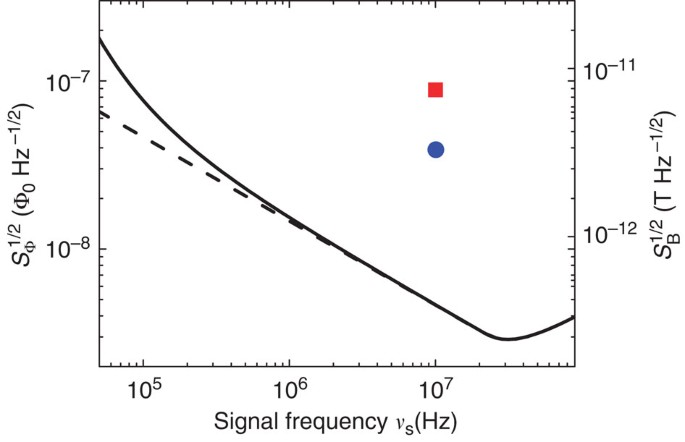Figure 5: Magnetic flux and field detection sensitivity for present results and ideal case.

In conclusion, we demonstrated a high-sensitivity magnetometer based on an artificial atom. The high magnetic field sensitivity combined with the micron spatial resolution is relevant to applications such as detection of electron spin resonance, scanning probe microscopy, and sensitive current and voltage amplifiers1. This detector is particularly interesting for exploring the dynamics of quantum systems at low temperatures with minimal backaction. The results here illustrate the potential that artificial quantum systems have for quantum sensing.

## Methods

### Device fabrication

The PCQ presented in this work is realized using a two-step fabrication process. In the first step, a resist layer is deposited on a silicon wafer and patterned using optical lithography to define all the device elements except the qubit. The resist is developed, then 200 nm of aluminium is evaporated onto the chip. Lifting off the resist removes Al from unpatterned regions. The second layer, which contains the qubit and the connexions to the central line of the coplanar waveguide resonator, is realized using standard shadow evaporation of aluminium. The Josephson junctions are formed by two aluminium layers, with thicknesses 40 and 65 nm, respectively, separated by an in situ grown thin aluminium oxide layer.

### Measurement set-up

The experiments are performed in a dilution refrigerator, at a temperature of 43 mK (see 39 for additional details). The device is placed inside a copper box, connected to a printed circuit board by wire bonding. Connexions to transmission lines are done using microwave launchers on the printed circuit board. Magnetic shielding is implemented using three layers of high magnetic permeability material. The sample is connected to room temperature electronics using coaxial cables, which include various filter, isolation and amplification sections. The signal at the output port of the resonator is amplified using a low-noise high-electron-mobility transistor amplifier with a noise temperature of 4 K.

Readout and control pulses are implemented using modulation of continuous-wave signals produced by synthesizers. Modulation signals are produced using arbitrary waveform generators with a time resolution of 1 ns (4 ns) for control (readout) pulses. The signal at the output of the resonator, amplified using the high-electron-mobility transistor amplifier, is further amplified using an amplification chain at room temperature, demodulated and digitized. The average of each readout output pulse is recorded for each repetition. The time series of the digital measurement output are used to extract average quantities and the noise power spectral density (PSD).

Magnetic field biasing of the qubit is performed using a centimetre size coil attached to the copper box and fed by a current produced by a high-stability current source.

### Calculation of detector output noise from experiments

The detector output noise, that is Sr and Sc for the two methods discussed in the text, respectively, is determined as follows. For a given detector pulse configuration and one given value of the Vac amplitude, Ns series of measurement results, with each series formed of Nm measurements, are acquired. The measurement results are denoted as rjk, with 1≤jNs and 1≤kNm. For each series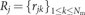we calculate the average r̄j. The average and standard deviation of the set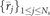, plotted in Fig. 3d versus Vac, are used to extract the transfer function (∂〈r〉)/(∂Vac). The spectral density of the r noise is calculated as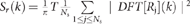, with T=NmTrep the total series time. We defined the discrete Fourier transform of the series Rj as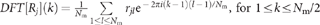, for 1≤kNm/2. The array Sr(k) gives the single-sided spectral density of the noise, corresponding to frequencies fk=k/(NmTrep). The Sc noise is determined in an analogous manner.

### Relation between detector total noise and quadrature noise

We consider a signal applied to the detector input of the form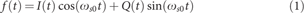with f the magnetic flux Φ normalized to the flux quantum Φ0. In Supplementary Methods, section ‘Two-quadrature detection’, we discuss two protocols which allow for the measurement of the two quadratues, I and Q, of the signal. Using suitable transfer functions, as expressed by Supplementary equations S14 and S15 in Supplementary Methods, one can calculate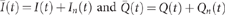, with I(t) and Q(t) the signal parts and In(t) and Qn(t) the noisy parts. The noise component represents noise due to measurement randomness and possibly other components. We take in the followingto be functions of time, although in the calculation of noise power spectra and Fourier components based on experiments, we use the discrete series of single measurement results. The two stochastic processes, In(t) and Qn(t), are stationary: the correlation functions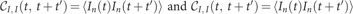, with 〈〉 representing a process ensemble average, only depend on the time t′. Stationarity is valid assuming that the measured fields are weak. Under the same assumption, the cross-correlation functions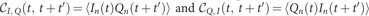also depend only on the time difference t′.

The measured parameter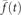calculated based on the measured quadratures can be written as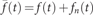. Here f(t) is the the signal defined in equation 1. The second part,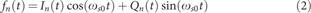is a random process. It is however not a stationary process, but a cyclostationary process40. The correlation function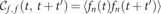is periodic with t, with a period in given by 2π/ωs0.

In general, for two processes A(t) and B(t) for which the correlation functiononly depends on the time difference t′, we define the cross-PSD as: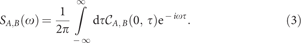When B is identical with A, this definition yields the regular PSD. For the process fn(t), which is not stationary, we consider the time averaged PSD. This is the relevant quantity when the noise is averaged over times long enough compared with the period 2π/ωs0 (ref. 41). The relation between the time averaged PSD Sf, f, and the PSD and cross-PSD for the processes In(t) and Qn(t) is given by: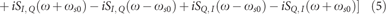where we omitted the subscript n for quantities In and Qn. All the cross-correlation spectral density functions vanish, as the two-quadrature measurement protocols discussed in the Supplementary Information Methods involve independent measurements. We next introduce the single-sided PSD for a random process A(t), defined for positive frequencies ω, as: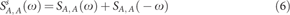For a frequency ω=ωs0+δ detuned from ωs0 by an amount δ with |δ|<ωs0, and assuming that the spectrum of the quadrature noise is contained in the band (−ωs0, ωs0), we obtain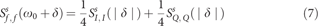On the basis of equation 7, we can determine the relation between the equivalent input and the quadrature noise measured in our experiment. For a measurement in which only one quadrature, for definitiveness assumed to be I, is measured with repetition time Trep, let us denote the noise by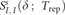. Consider next that the protocol is replaced by interleaved sampling (Supplementary Information Methods, section ‘Two-quadrature detection’). Now each quadrature is measured with a repetition time 2Trep. We have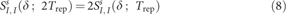because the same total noise is spread over a smaller bandwidth, 1/2Trep, as opposed to 1/Trep. We also assume that the Q quadrature is measured in the same way, hence the output noise is the same. Using equation 7 we derive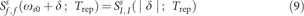The consequence of relation 9 for the interpretation of the data in our experiment is very important. It tells us that the equivalent total detector noise input is properly described by the quantity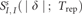, which is the quantity that we discuss in the paper.

How to cite this article: Bal, M. et al. Ultrasensitive magnetic field detection using a single artificial atom. Nat. Commun. 3:1324 doi: 10.1038/ncomms2332 (2012).

## References

1. 1

Clarke J., Braginski A. (eds.) The SQUID Handbook Vol 1,, (Wiley-VCH (2004).

2. 2

Budker D., Romalis M. Optical magnetometry. Nat. Phys. 3, 227–234 (2007).

3. 3

Dang H. B., Maloof A. C., Romalis M. V. Ultrahigh sensitivity magnetic field and magnetization measurements with an atomic magnetometer. Appl. Phys. Lett. 97, 151110 (2010).

4. 4

Degen C. L. Scanning magnetic field microscope with a diamond single-spin sensor. Appl. Phys. Lett. 92, 243111 (2008).

5. 5

Arcizet O. et al. A single nitrogen-vacancy defect coupled to a nanomechanical oscillator. Nat. Phys. 7, 879–883 (2011).

6. 6

Kolkowitz S. et al. Coherent sensing of a mechanical resonator with a single-spin qubit. Science 335, 1603–1606 (2012).

7. 7

Chang A. M. et al. Scanning hall probe microscopy. Appl. Phys. Lett. 61, 1974–1976 (1992).

8. 8

Forstner S. et al. Cavity optomechanical magnetometer. Phys. Rev. Lett. 108, 120801 (2012).

9. 9

Taylor J. et al. High-sensitivity diamond magnetometer with nanoscale resolution. Nat. Phys. 4, 810–816 (2008).

10. 10

Maze J. R. et al. Nanoscale magnetic sensing with an individual electronic spin in diamond. Nature 455, 644–647 (2008).

11. 11

Balasubramanian G. et al. Nanoscale imaging magnetometry with diamond spins under ambient conditions. Nature 455, 648–651 (2008).

12. 12

Mooij J. E. et al. Josephson persistent-current qubit. Science 285, 1036–1039 (1999).

13. 13

Clarke J., Wilhelm F. K. Superconducting quantum bits. Nature 453, 1031–1042 (2008).

14. 14

Il’ichev E., Greenberg Y. S. Flux qubit as a sensor of magnetic flux. Europhys. Lett. 77, 58005 (2007).

15. 15

Blais A., Huang R.-S., Wallraff A., Girvin S. M., Schoelkopf R. J. Cavity quantum electrodynamics for superconducting electrical circuits: an architecture for quantum computation. Phys. Rev. A 69, 062320 (2004).

16. 16

Wallraff A. et al. Strong coupling of a single photon to a superconducting qubit using circuit quantum electrodynamics. Nature 431, 162–167 (2004).

17. 17

Abdufarrukh A., Abdumalikov J., Astafiev O., Nakamura Y., Pashkin Y. A., Tsai J. Vacuum rabi splitting due to strong coupling of a flux qubit and a coplanar-waveguide resonator. Phys. Rev. B 78, 180502 (2008).

18. 18

Niemczyk T. et al. Circuit quantum electrodynamics in the ultrastrong-coupling regime. Nat. Phys. 6, 772–776 (2010).

19. 19

Wallraff A. et al. Approaching unit visibility for control of a superconducting qubit with dispersive readout. Phys. Rev. Lett. 95, 060501 (2005).

20. 20

Yoshihara F., Harrabi K., Niskanen A. O., Nakamura Y., Tsai J. S. Decoherence of flux qubits due to 1/f flux noise. Phys. Rev. Lett. 97, 167001 (2006).

21. 21

Bylander J. et al. Noise spectroscopy through dynamical decoupling with a superconducting flux qubit. Nat. Phys. 7, 565–570 (2011).

22. 22

Lupascu A. et al. Quantum non-demolition measurement of a superconducting two-level system. Nat. Phys. 3, 119–125 (2007).

23. 23

Picot T., Lupascu A., Saito S., Harmans C. J. P. M., Mooij J. E. Role of relaxation in the quantum measurement of a superconducting qubit using a nonlinear oscillator. Phys. Rev. B 78, 132508 (2008).

24. 24

Schuster D. I. et al. ac stark shift and dephasing of a superconducting qubit strongly coupled to a cavity field. Phys. Rev. Lett. 94, 123602 (2005).

25. 25

Awschalom D. D. et al. Low-noise modular microsusceptometer using nearly quantum limited dc squids. Appl. Phys. Lett. 53, 2108–2110 (1988).

26. 26

Wellstood F. C., Urbina C., Clarke J. Hot electron effect in the dc -squid. IEEE Trans. Magn. 25, 1001–1004 (1989).

27. 27

Koch R. H., Harlingen D. J. V., Clarke J. Quantum noise theory for the dc squid. Appl. Phys. Lett. 38, 380–382 (1981).

28. 28

Danilov V., Likharev K., Zorin A. Quantum noise in squids. IEEE Trans. Magnetics 19, 572–575 (1983).

29. 29

Kominis I., Kornack T., Allred J., Romalis M. A subfemtotesla multichannel atomic magnetometer. Nature 422, 596–599 (2003).

30. 30

Shah V., Knappe S., Schwindt P., Kitching J. Subpicotesla atomic magnetometry with a microfabricated vapour cell. Nat. Photon. 1, 649–652 (2007).

31. 31

Wildermuth S. et al. Bose-Einstein condensates: microscopic magnetic-field imaging. Nature 435, 440–440 (2005).

32. 32

Vengalattore M. et al. High-resolution magnetometry with a spinor bose-einstein condensate. Phys. Rev. Lett. 98, 200801 (2007).

33. 33

Wildermuth S. et al. Sensing electric and magnetic fields with bose-einstein condensates. Appl. Phys. Lett. 88, 264103 (2006).

34. 34

Lee S.-K., Sauer K. L., Seltzer S. J., Alem O., Romalis M. V. Subfemtotesla radio-frequency atomic magnetometer for detection of nuclear quadrupole resonance. Appl. Phys. Lett. 89, 214106 (2006).

35. 35

de Lange G., Ristè D., Dobrovitski V., Hanson R. Single-spin magnetometry with multipulse sensing sequences. Phys. Rev. Lett. 106, 080802 (2011).

36. 36

Castellanos-Beltran M. A., Irwin K. D., Hilton G. C., Vale L. R., Lehnert K. W. Amplification and squeezing of quantum noise with a tunable josephson metamaterial. Nat. Phys. 4, 929–931 (2008).

37. 37

Bergeal N. et al. Phase-preserving amplification near the quantum limit with a josephson ring modulator. Nature 465, 64–68 (2010).

38. 38

Wellstood F. C., Urbina C., Clarke J. Low-frequency noise in dc superconducting quantum interference devices below 1 k. Appl. Phys. Lett. 50, 772–774 (1987).

39. 39

Ong F. R., Orgiazzi J.-L., de Waard A., Frossati G., Lupascu A. Insertable system for fast turnaround time microwave experiments in a dilution refrigerator. Rev. Sci. Instrum. 83, 093904 (2012).

40. 40

Gardner W. A., Napolitano A., Paura L. Cyclostationarity: half a century of research. Signal Process 86, 639–697 (2006).

41. 41

Gardner W. A. Rice’s representation for cyclostationary processes. IEEE Trans. Commun. 35, 74–78 (1987).

## Acknowledgements

We are grateful to Mohammad Ansari, Jonathan Friedman, Jay Gambetta, Pieter de Groot, Seth Lloyd, Britton Plourde and Frank Wilhelm for discussions. We acknowledge support from NSERC through Discovery and RTI grants, Canada Foundation for Innovation, Ontario Ministry of Research and Innovation, and Industry Canada. A.L. is supported by a Sloan Fellowship and J.L.O. is supported by a WIN scholarship.

## Author information

Authors

### Contributions

M.B. and A.L. designed the experiment; M.B. fabricated the qubit/resonator device, conducted the experiment and analysed the data; J.L.O. and F.O. contributed to the development of the experimental set-up; C.D. contributed to the development of software for data acquisition; A.L. and M.B. wrote the manuscript; and all the authors discussed the data and commented on the manuscript.

### Corresponding author

Correspondence to A. Lupascu.

## Ethics declarations

### Competing interests

The authors declare no competing financial interests.

## Supplementary information

### Supplementary Methods

Supplementary Figures S1-S5, Supplementary Methods and Supplementary References (PDF 603 kb)

## Rights and permissions

Reprints and Permissions

Bal, M., Deng, C., Orgiazzi, JL. et al. Ultrasensitive magnetic field detection using a single artificial atom. Nat Commun 3, 1324 (2012). https://doi.org/10.1038/ncomms2332

• Accepted:

• Published:

• ### Phase estimation algorithm for the multibeam optical metrology

• V. V. Zemlyanov
• N. S. Kirsanov
• G. B. Lesovik

Scientific Reports (2020)

• ### Electron paramagnetic resonance spectroscopy using a single artificial atom

• Hiraku Toida
• Yuichiro Matsuzaki
• Shiro Saito

Communications Physics (2019)

• ### Heisenberg-limited single-mode quantum metrology in a superconducting circuit

• W. Wang
• Y. Wu
• L. Sun

Nature Communications (2019)

• ### High-sensitivity magnetometry with a single atom in a superposition of two circular Rydberg states

• E. K. Dietsche
• A. Larrouy
• S. Gleyzes

Nature Physics (2019)

• ### Quantum-enhanced magnetometry by phase estimation algorithms with a single artificial atom

• S. Danilin
• A. V. Lebedev
• G. S. Paraoanu

npj Quantum Information (2018)